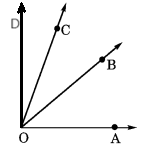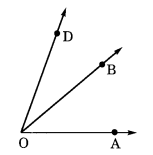NCERT Solutions for Class 6 Maths Chapter 4 Basic Geometrical Ideas Ex 4.3

# NCERT Solutions for Class 6 Maths Chapter 4 Basic Geometrical Ideas Ex 4.3

## NCERT Solutions for Class 6 Maths Chapter 4 Basic Geometrical Ideas Ex 4.3

NCERT Solutions for Class 6 Maths Chapter 4 Basic Geometrical Ideas Ex 4.3 are the part of NCERT Solutions for Class 6 Maths. Here you can find the NCERT Solutions for Class 6 Maths Chapter 4 Basic Geometrical Ideas Ex 4.3.

### Ex 4.3 Class 6 Maths Question 1.

Solution:
The angles in the given figure are:
(i)
DAB or A
(ii)
CBA or B
(iii)
DCB or C
(iv)

### Ex 4.3 Class 6 Maths Question 2.

(a) In the interior of DOE
(b) In the exterior of
EOF
(c) On
EOF

Solution:
(a) The point in the interior of DOE is A.
(b) The points in the exterior
EOF are A, C and D.
(c) The points on
EOF are B, E, O and F.

### Ex 4.3 Class 6 Maths Question 3.

Draw rough diagrams of two angles such that they have
(а) one point in common.
(b) two points in common.
(c) three points in common.
(d) four points in common.
(e) One ray in common.

Solution:
(a) In the following figure, O is the common point of AOB and COD.(b) In the following figure, O and B are the common points in
AOB and BOC.

(c) Such a diagram is not possible.
(d) Such a diagram is not possible.
(e) OB is the common ray of
AOB and BOD.You can also like these:

NCERT Solutions for Maths Class 7

NCERT Solutions for Maths Class 8

NCERT Solutions for Maths Class 9

NCERT Solutions for Maths Class 10

NCERT Solutions for Maths Class 11

NCERT Solutions for Maths Class 12